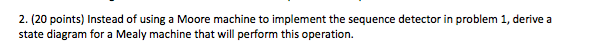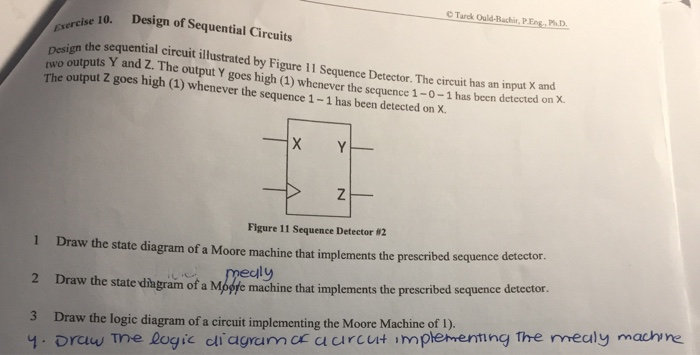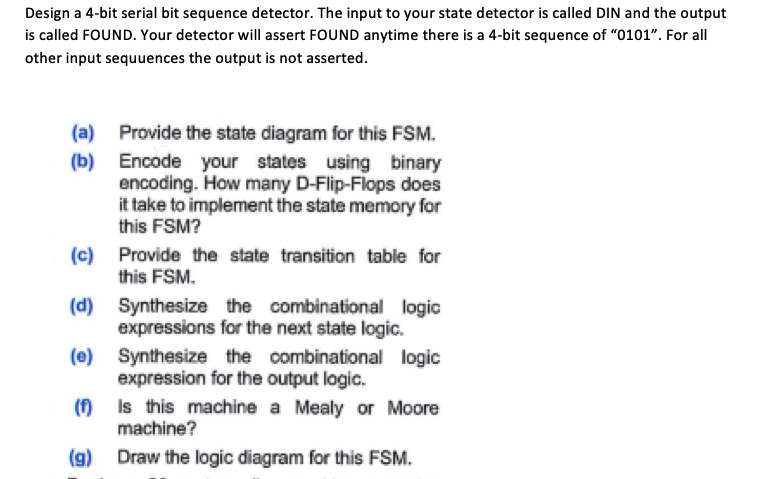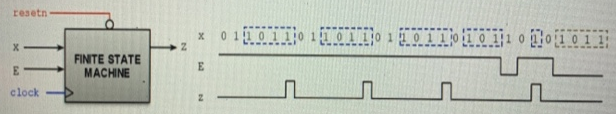Question

# Design a Moore Machine sequence (1 input and 1 output) detector circuit that recognizes the sequence...

Design a Moore Machine sequence (1 input and 1 output) detector circuit that recognizes the sequence “110” from an input stream. Draw the state diagram.If you have any doubts please comment.

Thank you...

#### Earn Coins

Coins can be redeemed for fabulous gifts.

Similar Homework Help Questions
• ### Design a MOORE FINITE STATE MACHINE for a Sequence Detector that detects sequentially the number 1510 in a stream of input bits

Design a MOORE FINITE STATE MACHINE for a Sequence Detector that detects sequentially the number 1510 in a stream of input bits. Label the input w. The output z is equal to 1 if the number 1510 was detected. After detecting the pattern (1510), the machine goes back in the initial state S0. a) Draw the state diagram for the FSM. Add an asynchronous Reset, active LOW. b) How many FFs do you need to implement this FSM? Note: Label the states S0,...

• ### 2. (20 points) Instead of using a Moore machine to implement the sequence detector in problem...2. (20 points) Instead of using a Moore machine to implement the sequence detector in problem 1, derive a state diagram for a Mealy machine that will perform this operation. 1. (20 points) For this problem, we want to design a circuit that checks for the input sequence 00101. Your circuit will have a one-bit input W and a one-bit output Z where Z-1 if the last five values of W observed on each positive edge of the clock are...

• ### Part 1: Design a Moore state machine that recognizes both a 1012 and a 0102 input...

Part 1: Design a Moore state machine that recognizes both a 1012 and a 0102 input pattern. This state machine has a 2- bit wide z output. If the 1012 pattern is detected, the state machine should output 102. If the 0102 pattern is detected, the state machine should output 012. In the initial state, the output should be 002 and in all other states, the output should be 112. Draw the state diagram and the state table. Part 2:...

• ### Draw a Moore-type state diagram and design a synchronous sequential circuit using D flip flops for...

Draw a Moore-type state diagram and design a synchronous sequential circuit using D flip flops for a 1-input/1-output "sequence detector" for the sequence 110 (be sure to recognize overlapping sequences). Draw the final circuit.

• ### please provide the answers of the 4 points thanks? C Tarek Ould-Bachir, PEng,PhD. Design of Sequential Circuits ise 10. nesign the sequential circuit illustrated by Figure 11 Sequence Detector. Th...please provide the answers of the 4 points thanks? C Tarek Ould-Bachir, PEng,PhD. Design of Sequential Circuits ise 10. nesign the sequential circuit illustrated by Figure 11 Sequence Detector. The cireuit has an input X and wo outputs Y and Z. The output Y goes high (1) whenever the sequence 1-0-1 has been detected on x. The output Z goes high (1) whenever the sequence 1-1 has been detected on X. Figure 11 Sequence Detector #2 1 Draw the state...

• ### Design a 4-bit serial bit sequence detector. The input to your state detector is called DIN...Design a 4-bit serial bit sequence detector. The input to your state detector is called DIN and the output is called FOUND. Your detector will assert FOUND anytime there is a 4-bit sequence of "0101". For all other input sequuences the output is not asserted. (a) (b) Provide the state diagram for this FSM. Encode your states using binary encoding. How many D-Flip-Flops does it take to implement the state memory for this FSM? (c) Provide the state transition table...

• ### Sequence detector: The machine has to generate z = 1 when it detects the sequence 1011Sequence detector: The machine has to generate z = 1 when it detects the sequence 1011. Once the sequence is detected, the circuit looks for a new sequence.  The signal E is an input enable: It validates the input x, i.e., if E = 1, x is valid, otherwise x is not valid. Draw the State Diagram (any representation), State Table, and the Excitation Table of this circuit with inputs E and x and output z. Is this a Mealy or a...

• ### Design a sequential circuit for a sequence detector that detects the sequence 10011

Design a sequential circuit for a sequence detector that detects the sequence 10011. A continuous bit stream is fed at the input of the circuit. Every time the circuit detects the sequence 10011, an output line is made HIGH a) Sketch Mealy FSM for the sequence detector. b) Tabulate the state table c) Using K-maps, write down the simplified Boolean expressions of the flip-flops input equations using T flip-flops d) Sketch the logic circuit diagram

• ### Draw a Moore-type state diagram and design a synchronous sequential circuit using D flip flops for...

Draw a Moore-type state diagram and design a synchronous sequential circuit using D flip flops for a 1-input/1-output "sequence detector" for the sequence 1001 (be sure to recognize overlapping sequences). Draw the final circuit.

• ### Design the Moore state diagram, state table, karnaugh map, and circuit diagram to check an input ...

Design the Moore state diagram, state table, karnaugh map, and circuit diagram to check an input stream and to produce an output Z=1 if the total number of zeros received is odd and the sequence 100 has occurred at least once. The total number of zeros received include before, during and after the sequence 100 is received. Use input test sequence X to the output sequence Z. X = 110100110010110 Z = 000001110110001